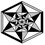# Difficult problem (for me at least) !!!!

If $f: [0,1] \rightarrow (0, \infty)$ such that $\int_0^1 f(x)\,dx = 1$, $\int_0^1 xf(x)\,dx = \alpha$ and $\int_0^1 x^2f(x)\,dx = \alpha^2$, then

A. $f(x)=5$

B. $f(2)=8$

C. $f(x)$ is not possible

D. $f(x)=2x-1$

I didn't post this as a problem because I am not sure whether the answer given in my book is correct or not. Please try to write an explanation rather than only the answer.Note by Maharnab Mitra
7 years, 5 months ago

This discussion board is a place to discuss our Daily Challenges and the math and science related to those challenges. Explanations are more than just a solution — they should explain the steps and thinking strategies that you used to obtain the solution. Comments should further the discussion of math and science.

When posting on Brilliant:

• Use the emojis to react to an explanation, whether you're congratulating a job well done , or just really confused .
• Ask specific questions about the challenge or the steps in somebody's explanation. Well-posed questions can add a lot to the discussion, but posting "I don't understand!" doesn't help anyone.
• Try to contribute something new to the discussion, whether it is an extension, generalization or other idea related to the challenge.

MarkdownAppears as
*italics* or _italics_ italics
**bold** or __bold__ bold
- bulleted- list
• bulleted
• list
1. numbered2. list
1. numbered
2. list
Note: you must add a full line of space before and after lists for them to show up correctly
paragraph 1paragraph 2

paragraph 1

paragraph 2

[example link](https://brilliant.org)example link
> This is a quote
This is a quote
    # I indented these lines
# 4 spaces, and now they show
# up as a code block.

print "hello world"
# I indented these lines
# 4 spaces, and now they show
# up as a code block.

print "hello world"
MathAppears as
Remember to wrap math in $$ ... $$ or $ ... $ to ensure proper formatting.
2 \times 3 $2 \times 3$
2^{34} $2^{34}$
a_{i-1} $a_{i-1}$
\frac{2}{3} $\frac{2}{3}$
\sqrt{2} $\sqrt{2}$
\sum_{i=1}^3 $\sum_{i=1}^3$
\sin \theta $\sin \theta$
\boxed{123} $\boxed{123}$

Sort by:

Consider the integral $\int_0^1 (x - \alpha)^2 f(x) \: dx.$

- 7 years, 5 months ago

no one of the A, B and D figures [0,1] to (0,+inf).

- 7 years, 5 months ago

@Jon Haussmann what is purpose of considering this integral.bcoz it is 0.as variance is 0.

- 7 years ago

answer isn't D. If we put x=0, (2x-1)=-1 which does not lie in the range. option A isn't possible. Putting f(x)=5 does not satisfy the 1st equation.

- 7 years ago

A is not true because assuming f(x) is 5 makes the first integral untrue.

B is not certainly true because without being able to directly solve for f(x), we have no idea what the f(2) should be; its out of the integral range

D is not true just by plugging it in again.

C must be the answer. Imagine f(x) and 1, x, and x^2 in perpendicular planes, and as you integrate the functions, you multiply f(x) and 1, x, or x^2, and a small dx to get a 3d volume. Between all of these volumes they all have the same height f(x), therefore the ratios of the volumes are simply the ratios of the widths of the solids. Since the ratio of ∫x^2dx/∫xdx is not equal to ∫xdx/∫dx (with 0,1 as limits), f(x) cannot exist

- 7 years ago

Multiply the first term by 4,second by -4,and third by 1 and add up those integrals.You will get RHS = 0 and LHS having integral of (alpha-2)^2*f(x) from 0 to 1. Now f(x) is positive and the other term is also positive so integral of a positive function can never be zero.So no function in possible.

- 7 years ago